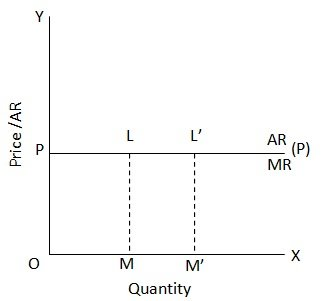Average Revenue

# Average Revenue Assignment Help

Home / Microeconomics Assignment Help / Average Revenue

# Average Revenue

Total Revenue shows the total sale proceeds of a firm. Average Revenue gives us the revenue per unit of the output sold and is obtained by dividing the total revenue with the number of units sold. Thus AR = TR/Q, where Q is the total quantity of the product sold in the market. Average revenue is to the producer what price per unit is to the consumer. Hence, AR = P. this can also be explained as follows:

We have already seen that AR = TR/Q.
But TR = P x Q
AR = P x Q/Q = P.Thus, average revenue is always equal to price. If price per unit of the goods remains the same, the AR curve is parallel to the horizontal axis at a distance which measures price on the vertical axis. This type of the AR curve. Whether the quantity sold is OM or OM’ the AR, which is LM and L’m’ respectively remains the same and equal to price OP.

However, generally, when quantity sold increases, the price per unit falls, as the consumers purchase a larger quantity only at a lower per unit price. Thus, the total revenue increases at a diminishing rate, i.e. it increases less than proportionate to the increase in quantity. Hence, AR (curve) shall be falling.

### Submit Homework

Submit your homework for a free quote